# Oscilloscope¶

You will be surprised that the EV3 text display can be tweaked to create an oscilloscope.

## The EV3 display¶

The EV3 has a 178 × 128 pixel Monochrome display. The corners have these coordinates:

• top-left - (1, 1)
• bottom-left - (1, 128)
• top-right - (178, 1)
• buttom-right - (178, 128)

It can display:

• 12.8 lines of small text
• 22 characters long

The small character occupy 10x8 pixels. One character is

• 9 pixels high
• 7 pixels wide

For exemple an A is composed of these pixels:

```1 2 3 4 5 6 7
x
x
x   x
x   x
x       x
x       x
x x x x x
x           x
x           x
```

## Characters used¶

For the oscilloscope we are going to use the vertical bar:

```1 2 3 4 5 6 7
x
x
x
x
x
x
x
x
x
```

the horizontal bar (underscore):

```1 2 3 4 5 6 7

x x x x x x x
```

## Display a horizontal line¶

With this information we are ready to display a horizontal line at position y. We define the variables x and y.

We write backwards. The reason for this is that the 7x9 pixel character is printed on a 8x10 pixel field. In fact the left and the top 1-pixel-wide line is erased.

So we initialize x to 178, and decrement by the caracter width of 7. With regards to y there is a -8 pixel offset.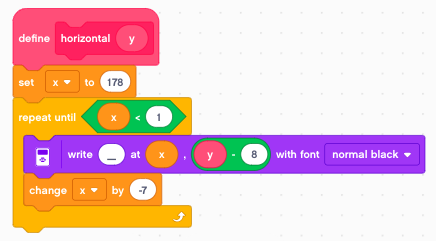## Display a vertical line¶

Then we define the vertical(x) function.

So we initialize y to 128, and decrement by the caracter height of 9. With regards to x there is a -4 pixel offset.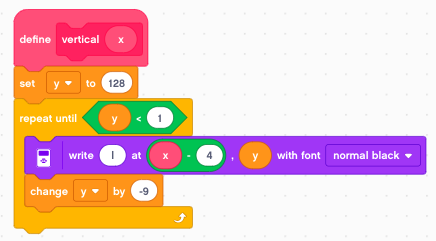## Display a grid¶

Finally we can draw a grid.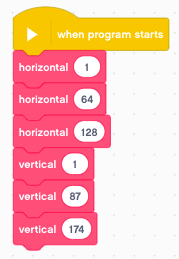## Draw a dot¶

The function write text at (x, y) can place a character at the position (x, y). To draw an arbitrary curve using dots, we could use the dot (`.`) or the hyphen (`-`).

But the best way is to use an underscore (`_`) followed by a space character. The space character is offset by 2 pixels and erases the 6 unused pixels of the underscore. This allows us to draw a dot every 2nd pixel.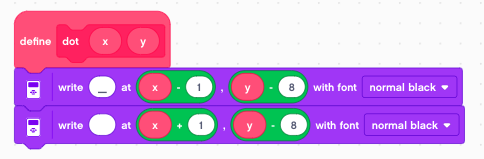The example below draws a diagonal line starting at (1, 1).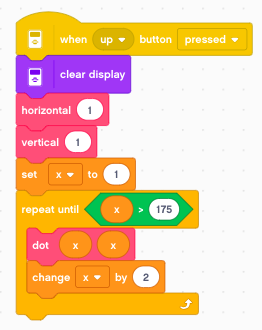## Display a scope trace¶

Now we have all the elements to program an oscilloscope. We start at x=1 and loop until x>175. At each iteration we increase x by 2.

The y value is the angular gyro velocity. We display it numerically on line 1. And we plot it with an offset of 64 to the screen.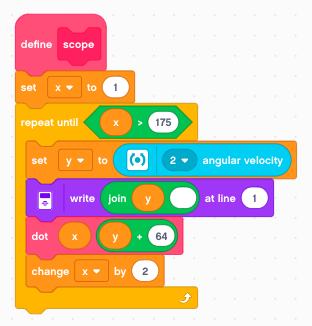With a button press we can acquire a single trace of 88 samples.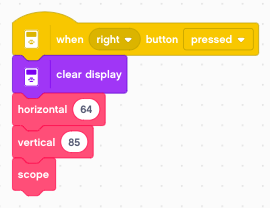## Measure continously¶

We can place it inside a loop and measure continously.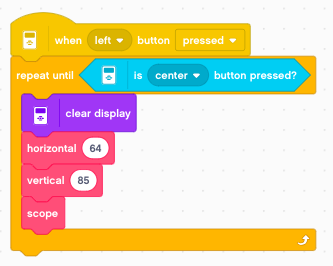You can download the programs so far: `scope.lmsp`